Clutch Prep is now a part of Pearson

# Charles Law (IGNORE)

See all sections
Sections
Pressure Units
The Ideal Gas Law
The Ideal Gas Law Derivations
The Ideal Gas Law Applications
Chemistry Gas Laws
Chemistry Gas Laws: Combined Gas Law
Mole Fraction
Partial Pressure
The Ideal Gas Law: Molar Mass
The Ideal Gas Law: Density
Gas Stoichiometry
Standard Temperature and Pressure
Effusion
Root Mean Square Speed
Kinetic Energy of Gases
Maxwell-Boltzmann Distribution
Velocity Distributions
Kinetic Molecular Theory
Van der Waals Equation
Boyle's Law (IGNORE)
Charles Law (IGNORE)
Ideal Gas Law (IGNORE)Jules Bruno

Charles’ Law, also known as the Law of Volumes, is a simple gas law that helps to explain what happens to the volume of a dry gas as it is heated.

Charles’ Law

Charles’ Law, named after the balloonist Jaques Charles, was theorized in order to examine the compressibility of gases. According to the findings of Charles, at constant pressure and amount for a gas, the volume (V) and absolute temperature (T) are directly proportional.

Thermal Expansion

The direct temperature-volume relationship with Charles’ Law can be illustrated by the following example: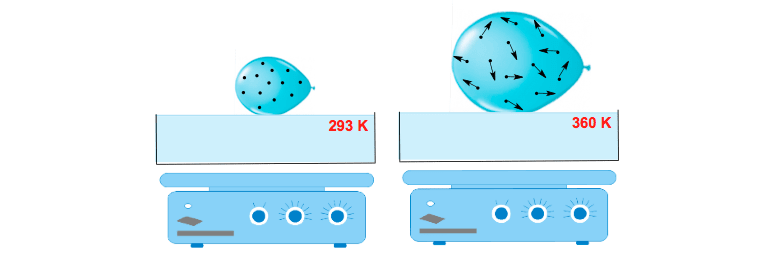Thermal Expansion of a ballon (Charles Law Application)

As the temperature from the hotplate is increased (290 K to 360 K), the gas molecules will absorb the additional thermal energy and convert it to kinetic energy. This causes the gas molecules to move faster and collide with the borders of the balloon with greater force. As a result of this increasing temperature, the volume inside the balloon also increases.

Temperature-Volume Plot

The linear relationship between temperature and volume can be plotted as the following graph: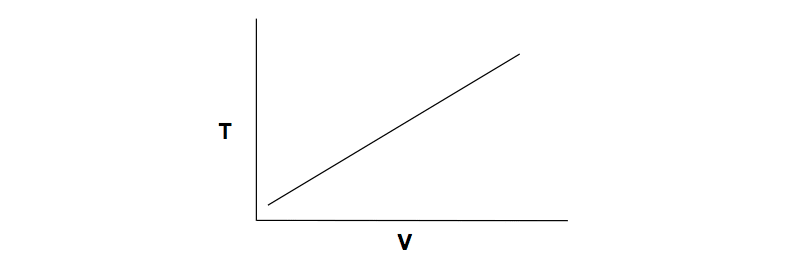Charles Law Graph Representation (VT Plot)

At constant pressure (P) and moles (n), this linear relationship between temperature (T) and volume (V) produces the following expression:Temperature-Volume Expression

Charles’ Law Formula

Isolating the variables of volume (V) and absolute temperature (T) can be accomplished by rearranging the Ideal Gas Law:Derivation of Charles' Law

When moles (n) and pressure (P) are in fixed states then the right side of the equation simplifies to:Temperature-Volume Ratio & k constant (Charles' Law)

When dealing with 2 Volumes (V1 and V2) and 2 Tempertaures (T1 and T2) we obtain Charles’ Law Formula:Charles' Law Formula

PRACTICE: A hot air balloon is filled with 1.33 x 106 L of an ideal gas on a cool morning (11°C) at 1.01 atm. The volume in the hot air balloon expands to 3.05 x 106 L. What is the temperature of the air in the balloon after its expansion? Assume that none of the gas escapes from the balloon and the conditions are isobaric.

STEP 1: Identify the variables that have changed and ignore the variables that have remained constant because they will not affect the final answer.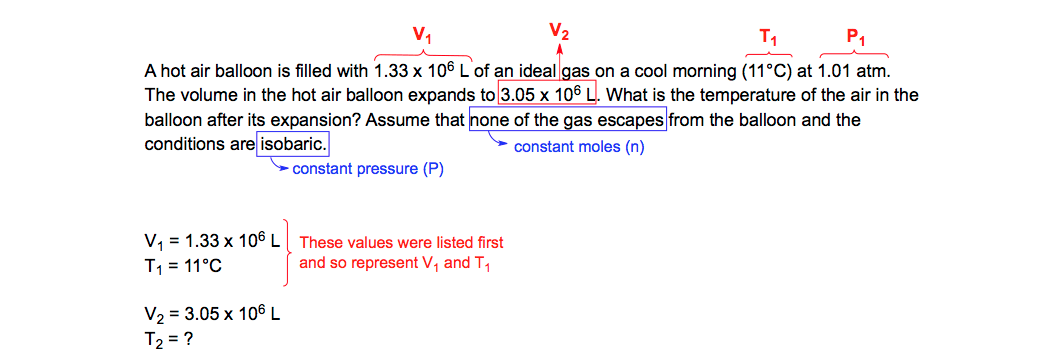Charles' Law (Identifying Variables)

STEP 2: Kinetic Molecular Theory states that all calculations dealing with temperature have to be done under absolute temperature. This means we must convert Celsius into Kelvin.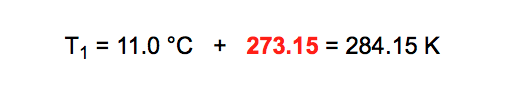Absolute Temperature (Charles' Law)

STEP 3: Plug the given values into the Charles’ Law formula.Charles' Law (V1/T1 = V2/T2)

STEP 4: Perform cross-multiplication between the two ratios.Charles' Law (Cross Multiplication)

STEP 5: Isolate the missing variable for the second temperature (T2).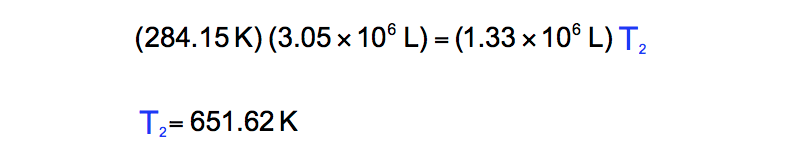Charles' Law (V1T2 = V2T1)

The Other Gas Law Equations

Charles’ Law represents one of the Simple Gas Laws in chemistry and like the others it tries to explain the reactive behavior of gases under varying conditions of pressure, volume, temperature or amount. The other Simple Gas Laws include Boyle’s Law, Avogadro’s Law and Gay-Lussac’s Law. Together these different laws combine to form the Ideal Gas Law when discussing ideal gases.Jules Bruno

Jules felt a void in his life after his English degree from Duke, so he started tutoring in 2007 and got a B.S. in Chemistry from FIU. He’s exceptionally skilled at making concepts dead simple and helping students in covalent bonds of knowledge.